# Sprite Sheet Maker

For LÖVE 0.10.2

Sprite Sheet Maker is by josefnpat and can be found here.

Tile-based video games are made up of a grid of tiles, where scenes are constructed from combining the images of a sprite sheet.

Sprite Sheet Maker takes an image made up of tiles, like this...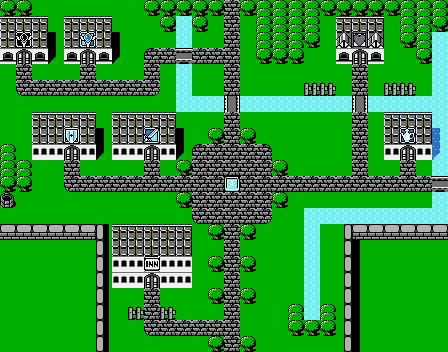... and makes a sprite sheet of all the unique tiles, like this!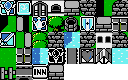It does this by:

• Looping through every tile-sized square of the input image (the tile size is predefined)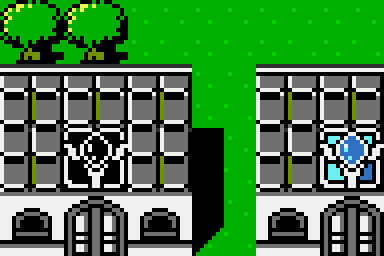• Looping through the pixels of this square.
• Creating a string based on the color values of all these pixels joined together.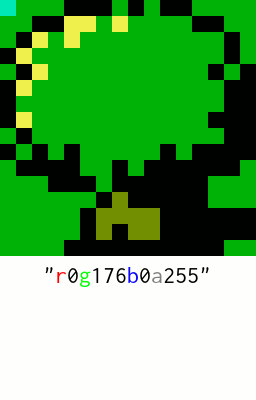• Storing the x and y position of the current tile in a table, with the key being the previously created string.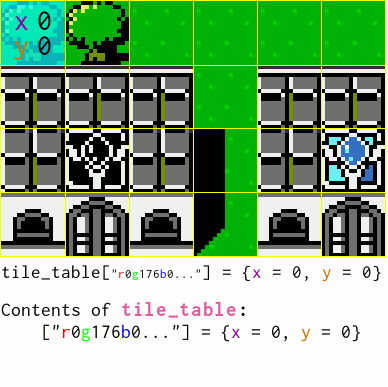• Creating a new ImageData for the output image.
• Looping through the table of unique tiles.
• Pasting from the stored positions of the input image to the output sprite sheet.
• Saving the output as an image file to the save directory (as defined in conf.lua).

It can also recognise tiles which have similar colours, but are not quite the same. This can be useful for input images which have JPEG artifacts.

Sprite Sheet Maker can take command-line arguments so you might want to run it from the command-line.

### Let's read Sprite Sheet Maker!

love.load can accept command-line arguments in the form of a table. For example, if LÖVE were to be run from the command-line like `love . test woo`...

`arg` would be `.`,
`arg` would be `test`, and
`arg` would be `woo`.

```function love.load(arg)
```

The first command-line argument (not including the name of the directory) is the name of the input file. If there are no command-line arguments, then input.gif is used by default.

The disjunction operator or returns its first argument if this value is not nil or false, otherwise it returns its second argument. If arg doesn't exist, i.e. it is nil, then input_filename is set to the second argument, input.gif.

```  input_filename = arg or "input.gif"
```

The output filename is the next argument, or output.png by default.

```  output_filename = arg or "output.png"
```

The width and height of each tile square is the next argument, otherwise the common tile size of 16 is used by default.

```  tile_size = arg or 16
```

The maximum number of tiles in each row of the output image is the next argument, or 8 by default.

```  spritesheet_row_width = arg or 8
```

pixel_value_reduction_factor is set to 2 to the power of the next argument, or 7 by default (that's 128), clamped between 1 and 255 by math.max (the maximum of 2 to the power of something and 1), and math.min (the minimum of this number and 255).

Every pixel value is divided by this variable, with any decimal places removed. In effect, this can make the pixels more similar.

As an example, let's say there were two pixels with red values of 213 and 217...

If pixel_value_reduction_factor had a value of `2^0` (which is 1), `213 / 1` and `217 / 1` would equal 213 and 217, so they would be considered different.

However, if pixel_value_reduction_factor had a value of `2^4` (which is 16), `213 / 16` and `217 / 16` (without decimal places) would both be equal to 13, and so these pixels would be considered to have the same red value!

Why is the number a power of 2? Because, as pixels have a maximum value of 255, it splits the value evenly (For example, if pixel_value_reduction_factor was 32, it would split the colour value into 8 even-ish parts. I think.)

Note that this won't actually change the image's colour, it will only affect the image detection.

```  pixel_value_reduction_factor = math.min(math.max(1, 2^(arg or 7)), 255)
```

love.filesystem.isFile returns true if the string it's given is a file, false otherwise.

```  if love.filesystem.isFile(input_filename) then
```

This is the ImageData of the input image.

ImageData is a LÖVE type to store images. It can be used to get and set pixel values, and save images to files, among other things. ImageDatas can't be drawn to the screen directly, but they can be converted to Images (using love.graphics.newImage), which can be.

ImageDatas can be created using love.image.newImageData in multiple ways. Here it is being created using the filename of an image file.

```    input = love.image.newImageData(input_filename)
```

If the image doesn't exist, an error is printed and the application is closed.

```  else
```

love.event.quit adds the "quit" event to the event queue.

```    love.event.quit()
```

love.run is LÖVE's main function, containing the main loop. In the default love.run function, love.load is run before any events are handled, including the "quit" event. To quit the program at this position in love.load it's also necessary to jump out of the love.load function by using return.

```    return
end
```

ImageDatas have methods getWidth and getHeight.

```  tiles_wide = input:getWidth() / tile_size
tiles_high = input:getHeight() / tile_size
```

These two variables will be either true or false depending on whether the image is exactly divisble by the tile size.

math.floor returns a "rounded down" version of the number it is given, for example `math.floor(123.789)` will return `123`. If tiles_wide/tiles_high are whole numbers (i.e. with no decimal places) then these variables will equal true, otherwise they will be false.

```  image_is_divisible_by_width = tiles_wide == math.floor(tiles_wide)
image_is_divisible_by_height = tiles_high == math.floor(tiles_high)
```

If either the image isn't divisible by width or by height, error message(s) are displayed the program exits.

```  if not image_is_divisible_by_width or not image_is_divisible_by_height then
if not image_is_divisible_by_width then
print("Error: Image width is not divisible by "..tile_size)
end
if not image_is_divisible_by_height then
print("Error: Image height is not divisible by "..tile_size)
end
love.event.quit()
return
else
print("Image is "..tiles_wide.." tiles wide.")
print("Image is "..tiles_high.." tiles high.")
end
```

tile_table will store the x and y grid positions of the unique tiles.

```  tile_table = {}
```

This program's main loop gets the pixel value for every pixel of the input image. The total number of "cycles" this loop does is equal to the number of pixels in the image, which can be found by multiplying the image's width by its height. The number of pixels in the image divided by 100 will be the amount of cycles completed for every 1 percent.

```  cycles_per_one_percent = input:getWidth() * input:getHeight() / 100
```

The cycles are counted using cycle_counter, which is reset every cycles_per_one_percent

```  cycle_counter = 0
```

The percentage which is displayed is stored in percentage_complete.

```  percentage_complete = 0

print("Building tile table...")
```

Instead of using print to display the output, io.write is used instead. A difference between them is that io.write doesn't put a new line after the printed string.

```  io.write("0%")
```

These first two loops are used to loop over every tile of the input image.

These loops have a maximum of one less than the value of their variables because they start at 0, and they start at 0 because it makes calculating the position of pixels a bit simpler, as pixel positions start at 0.

```  for tile_x = 0, tiles_wide - 1 do
for tile_y = 0, tiles_high - 1 do
```

tile_key is the string which will be the key for tile_table. Here it is created/reset.

```      tile_key = ""
```

Now for looping through each pixel!

```      for pixel_x = 0, tile_size - 1 do
for pixel_y = 0, tile_size - 1 do
```

1 is added to cycle_counter every cycle, and if it's past one percent then it's reset and percentage_complete is updated.

```          cycle_counter = cycle_counter + 1
if cycle_counter >= cycles_per_one_percent then
cycle_counter = 0
percentage_complete = percentage_complete + 1
```

\r is the escape sequence for a carriage return. This means that the display will start from the start of the line, overwriting what was previously there.

```            io.write("\r"..percentage_complete.."%")
end
```

getPixel is a method of ImageData, which given x and y positions, returns the red, green, blue, and alpha values of the pixel at that position.

Pixel coordinates (and display coordinates) in LÖVE start at 0.

```          r, g, b, a = input:getPixel(tile_x * tile_size + pixel_x,
tile_y * tile_size + pixel_y)
```

The colour values of the pixel get concatinated onto the end of tile_key.

The values are divided by pixel_value_reduction_factor, and math.floor removes any decimal places.

The reason the strings "r", "g", "b", and "a" are also being concatinated is so that the colour values are split up, otherwise `239841` could mean `r239g8b4` or `r23g98b31` or something else!

```          tile_key = tile_key.."r"..math.floor(r/pixel_value_reduction_factor)..
"g"..math.floor(g/pixel_value_reduction_factor)..
"b"..math.floor(b/pixel_value_reduction_factor)..
"a"..math.floor(a/pixel_value_reduction_factor)
end
end
```

The entry of tile_table with the key tile_key is set to a table with x and y keys containing the x and y grid positions of the tile. If there is already an entry with the same key as tile_key, then it is overwritten.

```      tile_table[tile_key] = {x = tile_x, y = tile_y}
end
end
```

print is used here because now we want a new line. Alternatively, this could be written using io.write with the new line escape sequence at the end, like `io.write("\r100\n")`.

```  print("\r100%")
```

The number of tiles in `tile_table` are added up. The length operator (#) used like `#tile_table` won't work because it is only useful for numerical arrays, as opposed to tables with keys.

```  unique_tiles = 0
for _, _ in pairs(tile_table) do
unique_tiles = unique_tiles + 1
end

print("Unique tiles: "..unique_tiles)
```

This variable stores the height (in tiles) of the columns in the sprite sheet output image.

For example, imagine a sprite sheet which has a total of 3 tiles and a row width of 2 tiles. It would therefore have a height of 2 tiles.

math.ceil returns the number it is given "rounded up". In the example above, `math.ceil(3 / 2)` would return 2, the required column height.

```  spritesheet_column_height = math.ceil(unique_tiles / spritesheet_row_width)
```

`spritesheet_row_width * tile_size` and `spritesheet_column_height * tile_size` return the width/height of the output sprite sheet in pixels.

```  print("Creating spritesheet: "..
spritesheet_row_width..
"x"..
spritesheet_column_height..
" ("..
spritesheet_row_width * tile_size..
"x"..
spritesheet_column_height * tile_size..
")")
```

A new, blank ImageData is made for the output. This time it's created using two numbers for its width and height.

```  spritesheet = love.image.newImageData(spritesheet_row_width * tile_size,
spritesheet_column_height * tile_size)
```

Now for pasting the tiles into the output sprite sheet!

spritesheet_row and spritesheet_column track the current row/column to paste to.

```  spritesheet_row = 0
spritesheet_column = 0
```

tile_table is looped through using a generic for loop with pairs.

The values of tile_table, which are tables storing the x and y grid positions of each unique tile, are given the variable name tile.

```  for _, tile in pairs(tile_table) do
```

ImageData's paste method copies a part of another ImageData to itself. The first argument is the ImageData to copy from.

The second and third arguments are the positions on the x and y axis to paste to.

spritesheet_row / spritesheet_column multiplied by tile_size are the row and column position in pixels.

The fourth and fifth arguments are the x and y positions of the source file to paste from.

tile.x and tile.y multiplied by tile_size will be the x and y positions (in pixels) to paste from.

The last two arguments are the width and height of what is pasted.

```    spritesheet:paste(input,
spritesheet_row * tile_size,
spritesheet_column * tile_size,
tile.x * tile_size,
tile.y * tile_size,
tile_size, tile_size)
```

After it's pasted, the row position is moved along.

```    spritesheet_row = spritesheet_row + 1
```

If the next row is equal to the row width, it's reset and the column number is increased. The reason why it's `spritesheet_row == spritesheet_row_width` and not `spritesheet_row > spritesheet_row_width` is because spritesheet_row starts at 0, not 1.

```    if spritesheet_row == spritesheet_row_width then
spritesheet_row = 0
spritesheet_column = spritesheet_column + 1
end
end
```

ImageData's encode method saves it as a file with the filename it is given.

```  spritesheet:encode(output_filename)
```

Yay!

```  print("Done!")

image = love.graphics.newImage(spritesheet)
```

The display size is set to the size of the image. love.window.setMode's first two arguments are the width and height of the display. Images have the methods getWidth and getHeight, which return their width and height respectively.

```  love.window.setMode(image:getWidth(), image:getHeight())
end
```

The image is drawn at the top left corner of the screen.

```function love.draw()
love.graphics.draw(image)
end
```

If escape is pressed, exit. Note that this won't take effect while the program is actually making the sprite sheet, it will only work after the image is displayed. This is because everything in love.load happens before key presses are handled.

```function love.keypressed(key)
if key == 'escape' then
love.event.quit()
end
end
```

And that's it! ^^

HTML generated by Locco.

Copyright is waived on all original parts of this code walkthrough.